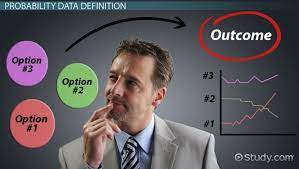Since the start of business school, I have had no second thoughts about the program until my adversary forced his way in. Mathematics showed up 😱. Just like that, the data analytics course took a quick turn. It went from excel sheets to my primary school days. Immediately, horror images of WAEC and Jamb started flashing in my head. I repeatedly said in my head, “I thought I was done with you.” To add to the confusion, the area of mathematics being discussed was probability. That was an area I never understood. For the first 30 minutes of that class, I was blank. To save my millions, I did a quick reset and reprogrammed my brain. Anyway, let me stop whining. Allow me to share some of what I learned with you. You know, it is all about the learning for me.

## What is probability?

Probability is a numerical measure of the likelihood that an event will occur. It is always assigned on a scale of 0 to 1. A probability near zero indicates that an event is unlikely to occur. A probability near one indicates that an event is almost certain to occur. There are many real-life situations in which we may have to predict the outcome of an event. We may be sure or not be sure of the results of an event. In such cases, we say that there is a probability of this event occurring or not occurring.

## Why probability?

To be a decision maker, one has to understand probability because it helps quantify the unknown. It aids decision making by telling us what is likely or unlikely. A way to put some order into things for insight. It is important in analytics. In the business world, you have to make plans based on assumptions, guesses and estimates. In decision making, the decision maker can be in a situation of risk or uncertainty.

• Risk is the situation under which the decision outcomes and their probabilities of occurrence are known to the decision-maker.
• Uncertainty is the situation under which the possible outcome or probability of an event is not available to the decision-maker.

## Random or statistical experiment

A random experiment is an action or process that leads to one of many possible outcomes. Examples:

• Experiment: Flip a coin         Outcomes: Heads or tails
• Experiment: Roll a die           Outcomes: 1, 2, 3, 4, 5, 6

The list of possible outcomes of a random experiment must be exhaustive and mutually exclusive.

## Events

An event is a collection or set of one or more simple events in a sample space.

Example: Roll of a Die  D = {1, 2, · · · , 6}

## The rules of assigning probability

• Rule 1: The probability of an impossible event is zero; the probability of a certain event is one.
• Rule 2: That is the sum of all the probabilities for all possible events or experiments must be equal to one. It is exhaustive.
• Rule 3: The probability of each experimental outcome must be between 0 and 1, inclusively.

## Assigning probabilities

There are three ways to assign probabilities to events: the classical approach, the relative-frequency approach, and the subjective approach.

• The classical method: used when an outcome is assumed to have an equal probability of occurrence.
• The relative frequency method: it is the number of times that the event occurs during experimental trials, divided by the total number of trials conducted.
• A subjective method: anyone’s personal opinion or judgment about whether a specific outcome is likely to occur. It contains no formal calculations and only reflects the subject’s opinions and past experience. It is often the best you can do when you have no past experience (so you cannot use relative frequency) and no theory (so you cannot use theoretical probability). An example of subjective probability is a “gut instinct” when making a trade.

That’s it for my key takeaways. In all, the class was not as bad as I thought. After listening to the recording, I felt at ease with probability. Right now, I am on a mission to learn probability. No matter what it takes, math and I are going to fall in love. At least for now, hehe!## Transforming Nigeria’s Local Government System for Rural Economic Growth and Increased GDP

in## PROBABILITY – A TOOL FOR DECISION MAKING

in